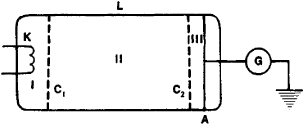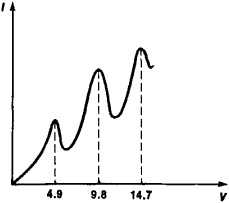# Franck-Hertz Experiment

Also found in: Wikipedia.

## Franck-Hertz experiment

[¦fräŋk ′hərts ik‚sper·ə·mənt]
(electronics)
Experiment for measuring the kinetic energy lost by electrons in inelastic collisions with atoms; it established the existence of discrete energy levels in atoms, and can be used to determine excitation and ionization potentials.

## Franck-Hertz Experiment

an experiment that provided experimental proof of the discreteness of the intrinsic energy of the atom. The experiment, a schematic diagram of which is given in Figure 1, was set up in 1913 by James Franck and Gustav Hertz.

A potential difference V, which accelerates electrons, is applied to the cathode K and grid C1 of an electron tube filled with mercury vapors, and the dependence of the current strength l on V is taken. A decelerating potential difference is applied to the grid C2 and the anode A. The electrons accelerated in region I undergo collisions with mercury atoms in region II. If the electron energy following the collision is sufficient to overcome the decelerating potential in region III, the electrons will strike the anode. The readings of galvanometer G therefore depend on the energy loss of the electrons during the collision.Figure 1. Schematic diagram of the Franck-Hertz experiment: (L) vessel containing mercury vapors at a pressure of 1 mm Hg, (K) heated cathode, (C1) and (C2) accelerating and decelerating grids, (A) anode, and (G) galvanometer for recording the current

In the course of the experiment, a monotonic increase in / was observed as the accelerating potential increased to 4.9 volts; that is, electrons with an energy E < 4.9 electron volts (eV) underwent elastic collisions with mercury atoms, and the intrinsic energy of the atoms did not change. Sharp decreases in the current (Figure 2) appeared at the value V = 4.9 volts (and at the multiple values 9.8 volts and 14.7 volts). This definitely indicated that at these values of V the collisions of the electrons with the atoms are inelastic, that is, the electron energy is sufficient to excite mercury atoms. At energy values that are multiples of 4.9 eV, electrons can undergo inelastic collisions several times. It thus was proved that the energy of an atom changes discontinuously rather than continuously, and that E = 4.9 eV is the smallest amount of energy that can be absorbed by the mercury atom in the ground state.Figure 2. Current strength as a function of the accelerating potential l(V) in the Franck-Hertz experiment

A. Compton, who repeated the Franck-Hertz experiment in 1922–23, found that when V > 4.9 volts, mercury vapors begin emitting light with a frequency v = ΔE/h, where ΔE = 4.9 eV and h is Planck’s constant. Mercury atoms excited by an electron collision thus emit a photon with an energy of 4.9 eV and return to the ground state.

### REFERENCE

Shpol’skii, E. V. Atomnaia fizika, 6th ed., vols. 1–2. Moscow, 1974.

A. V. KOLPAKOV

Site: Follow: Share:
Open / Close